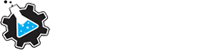Choose languageChoose language
VALIDATION, UNCERTAINTY AND
QUALITY CONTROL

## ConfLab Validation

The ConfLab Validation software performs many calculations, considering different validation parameters, focusing on the compliance of references for method validation and the new ISO 17025: 2017. The idea is that the user only enters with the experimental values and the software, automatically, calculates the parameters, presents the results and allows the printing of a fully customizable validation report, with all the traceability data required by the quality management systems . It is also compatible and meets the requirements of Good Laboratory Practices (GLP). Among the parameters for the Validation of Methods, is highlighted:
• Precision (Repeatability)
• Intermediate Precision
• Accuracy
• Accuracy with Fortified Samples
• Accuracy with Use of Reference Material
• Linearity
• Homoscedasticity Evaluation
• Regression of Ordinary Least Squares (MMQO) and Weighted Least Squares Regression (MMQP)
• General Testing
• Normality
• Independence
• Anova
• Significance of Intercept
• Absolute residue
• Relative residue
• Outliers (Grubbs by Level)
• Outliers (Total Grubbs)
• Accuracy
• Coefficient of variation
• Regression of Ordinary Least Squares (MMQO) Versus Regression of Weighted Minimum Least Squares (MMQP)
• LOD and LOQ
• Theoretical Calculations - White Sample standard deviations (or lower quantifiable level) - IUPAC / INMETRO
• Theoretical Calculations - White Sample standard deviations (or lower quantifiable level) - FDA / ANVISA
• Theoretical Calculations - Standard Deviation of Signal Intercept - FDA / ANVISA
• Experimental Calculations
• Matrix Effect
• Differences Between Calibration Curves
• Deviations from FMNs
• Robustness
• Youden's TestValidation, uncertainty and quality control